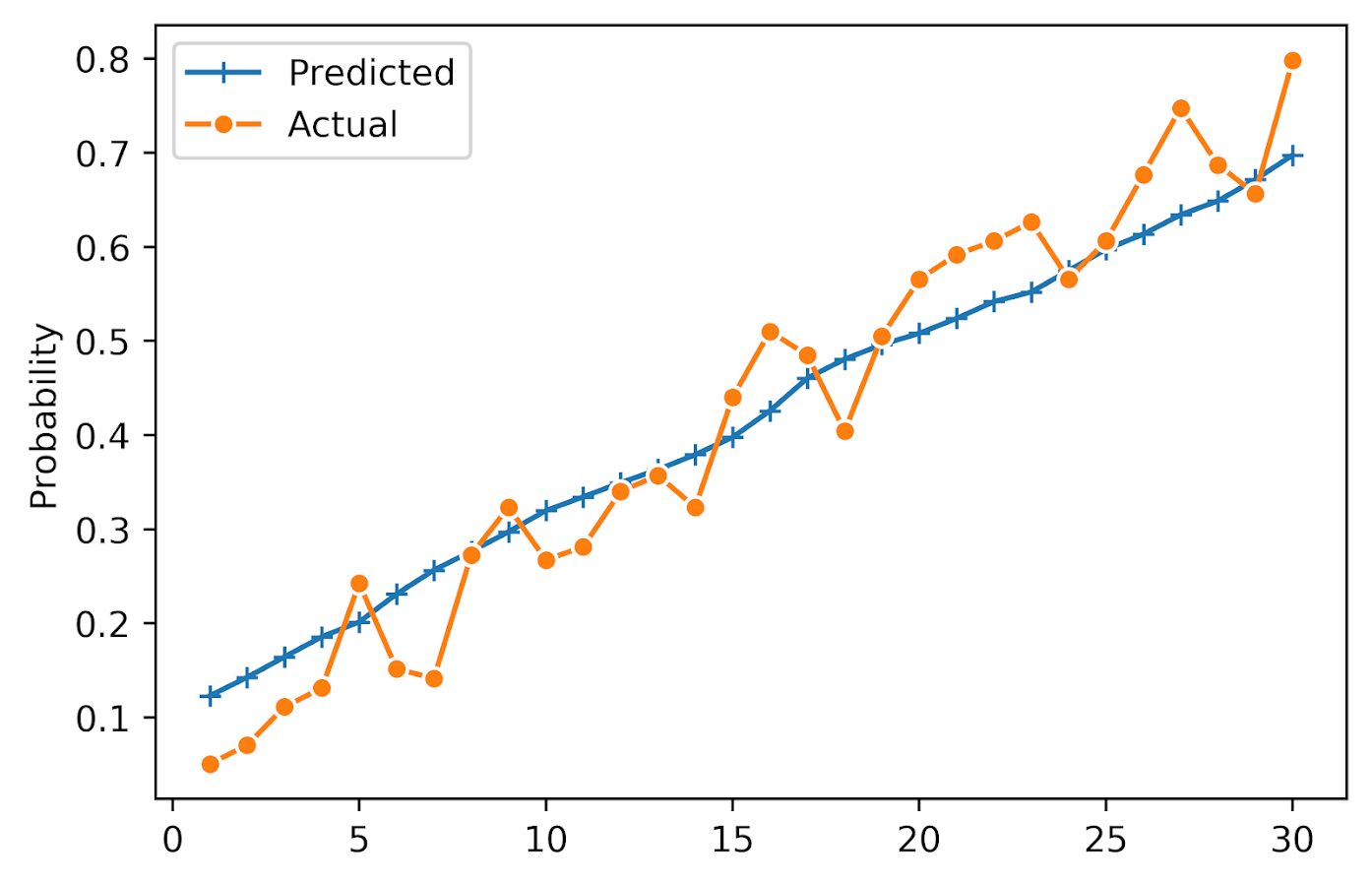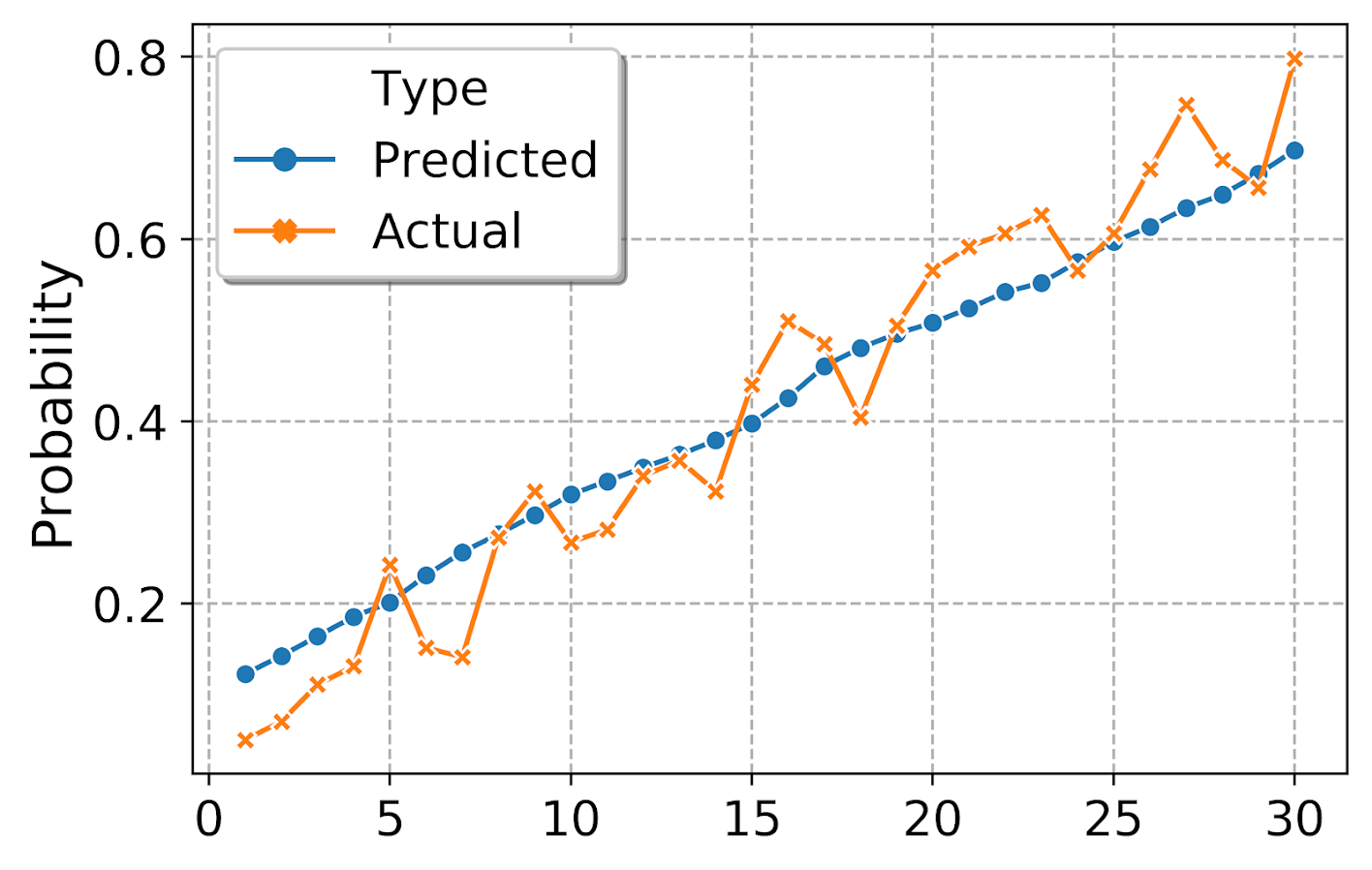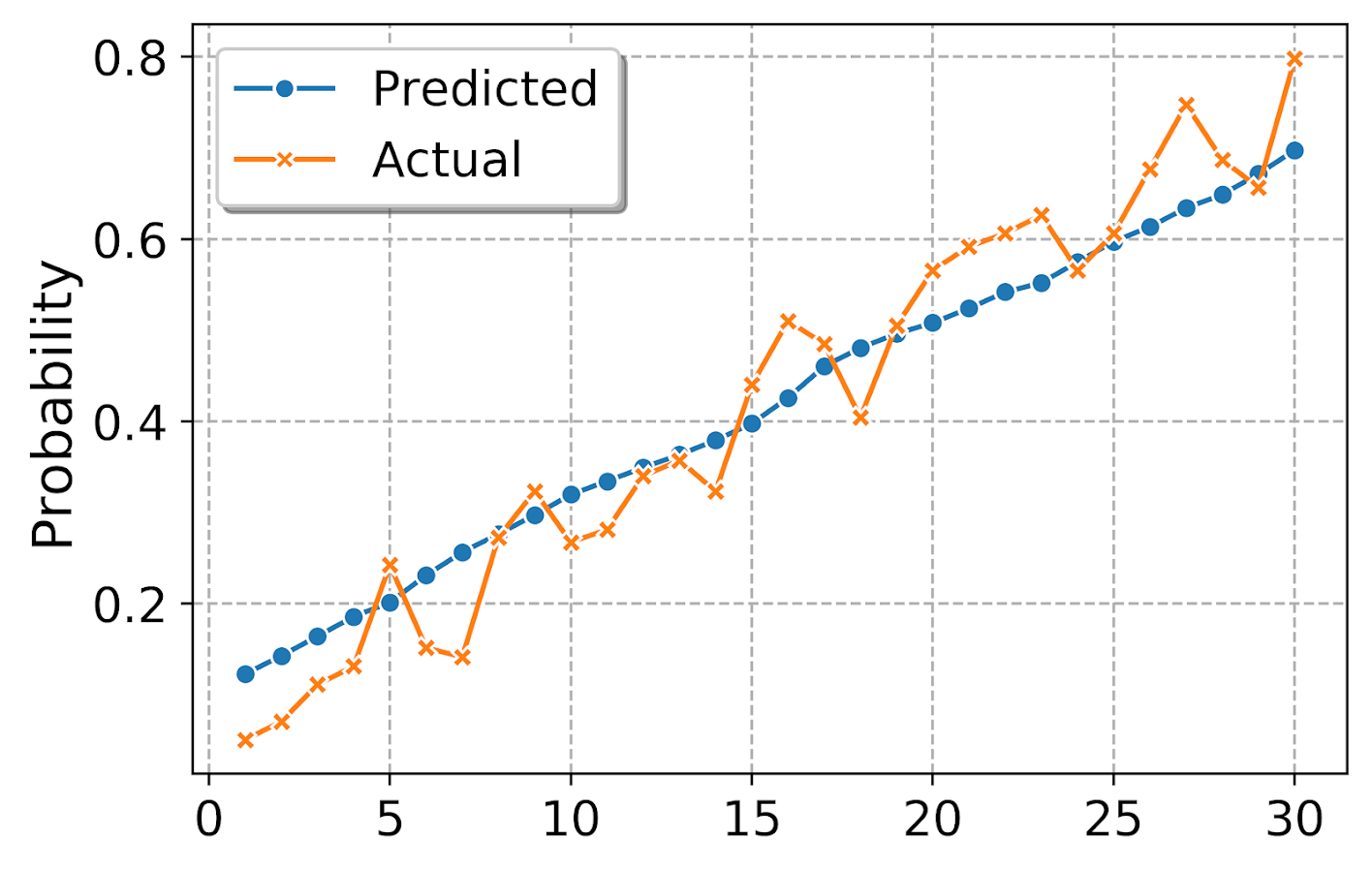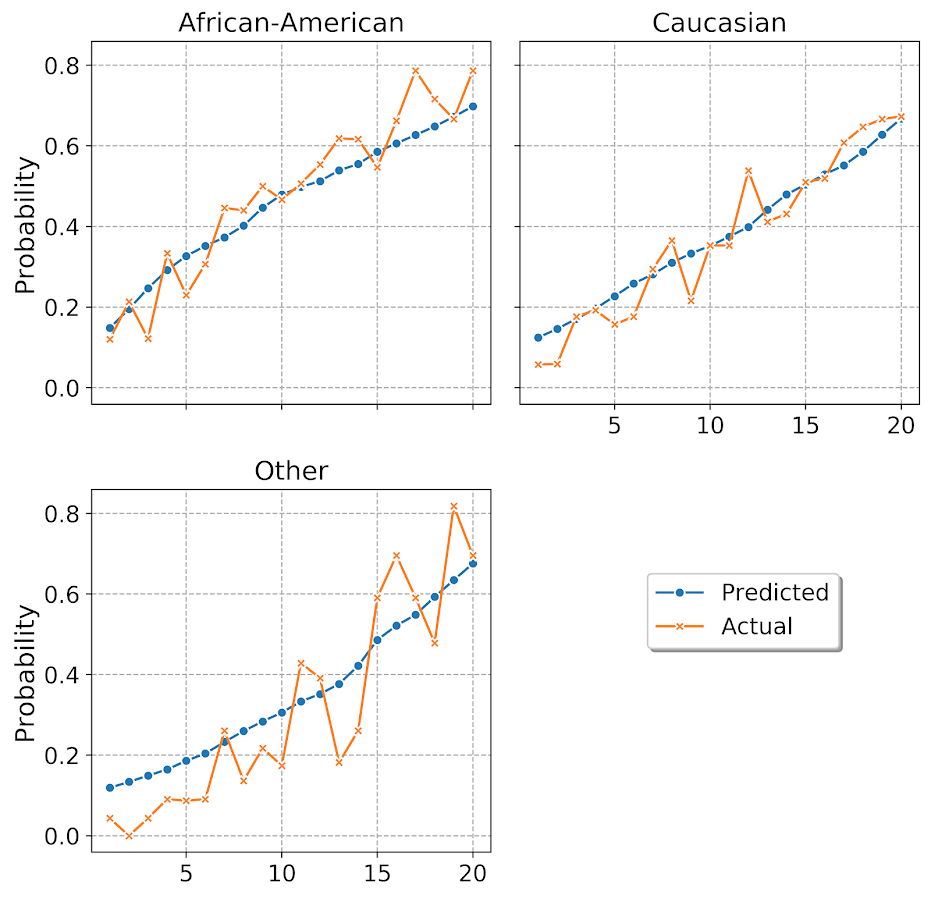# Notes on matplotlib and seaborn charts (python)

My current workplace is a python shop. I actually didn’t use pandas/numpy for most of my prior academic projects, but I really like pandas for data manipulation now that I know it better. I’m using python objects (lists, dictionaries, sets) inside of data frames quite a bit to do some tricky data manipulations.

I do however really miss using ggplot to make graphs. So here are my notes on using python tools to make plots, specifically the matplotlib and seaborn libraries. Here is the data/code to follow along on your own.

# some set up

First I am going to redo the data analysis for predictive recidivism I did in a prior blog post. One change is that I noticed the default random forest implementation in sci-kit was prone to overfitting the data – so one simple regularization was to either limit depth of trees, or number of samples needed to split, or the total number of samples in a final leaf. (I noticed this when I developed a simulated example xgboost did well with the defaults, but random forests did not. It happened to be becauase xgboost defaults had a way smaller number of potential splits, when using similar defaults they were pretty much the same.)

Here I just up the minimum samples per leaf to 100.

``````#########################################################
#set up for libraries and data I need
import pandas as pd
import os
import numpy as np
from sklearn.ensemble import RandomForestClassifier
import matplotlib
import matplotlib.pyplot as plt
import seaborn as sns

my_dir = r'C:\Users\andre\Dropbox\Documents\BLOG\matplotlib_seaborn'
os.chdir(my_dir)

#Modelling recidivism using random forests, see below for background
#https://andrewpwheeler.com/2020/01/05/balancing-false-positives/

#Preparing the variables I want
recid_prep = recid[['Recid30','CompScore.1','CompScore.2','CompScore.3',
recid_prep['Male'] = 1*(recid['sex'] == "Male")
recid_prep['Fel'] = 1*(recid['c_charge_degree'] == "F")
recid_prep['Mis'] = 1*(recid['c_charge_degree'] == "M")
recid_prep['race'] = recid['race']

#Now generating train and test set
recid_prep['Train'] = np.random.binomial(1,0.75,len(recid_prep))
recid_train = recid_prep[recid_prep['Train'] == 1]
recid_test = recid_prep[recid_prep['Train'] == 0]

#Now estimating the model
ind_vars = ['CompScore.1','CompScore.2','CompScore.3',
dep_var = 'Recid30'
rf_mod = RandomForestClassifier(n_estimators=500, random_state=10, min_samples_leaf=100)
rf_mod.fit(X = recid_train[ind_vars], y = recid_train[dep_var])

#Now applying out of sample
pred_prob = rf_mod.predict_proba(recid_test[ind_vars] )
recid_test['prob'] = pred_prob[:,1]
#########################################################``````

# matplotlib themes

One thing you can do is easily update the base template for matplotlib. Here are example settings I typically use, in particular making the default font sizes much larger. I also like a using a drop shadow for legends – although many consider drop shadows for data chart-junky, they actually help distinguish the legend from the background plot (a trick I learned from cartographic maps).

``````#########################################################
#Settings for matplotlib base

andy_theme = {'axes.grid': True,
'grid.linestyle': '--',
'legend.framealpha': 1,
'legend.facecolor': 'white',
'legend.fontsize': 14,
'legend.title_fontsize': 16,
'xtick.labelsize': 14,
'ytick.labelsize': 14,
'axes.labelsize': 16,
'axes.titlesize': 20,
'figure.dpi': 100}

print( matplotlib.rcParams )
#matplotlib.rcParams.update(andy_theme)

#print(plt.style.available)
#plt.style.use('classic')
#########################################################``````

I have it commented out here, but once you define your dictionary of particular style changes, then you can just run `matplotlib.rcParams.update(your_dictionary)` to update the base plots. You can also see the ton of options by printing `matplotlib.rcParams`, and there are a few different styles already available to view as well.

# creating a lift-calibration line plot

Now I am going to create a plot that I have seen several names used for – I am going to call it a calibration lift-plot. Calibration is basically “if my model predicts something will happen 5% of the time, does it actually happen 5% of the time”. I used to always do calibration charts where I binned the data, and put the predicted on the X axis, and observed on the Y (see this example). But data-robot has an alternative plot, where you superimpose those two lines that has been growing on me.

``````#########################################################
#Creating a calibration lift-plot for entire test set

bin_n = 30
recid_test['Bin'] = pd.qcut(recid_test['prob'], bin_n, range(bin_n) ).astype(int) + 1
recid_test['Count'] = 1

agg_bins = recid_test.groupby('Bin', as_index=False)['Recid30','prob','Count'].sum()
agg_bins['Predicted'] = agg_bins['prob']/agg_bins['Count']
agg_bins['Actual'] = agg_bins['Recid30']/agg_bins['Count']

#Now can make a nice matplotlib plot
fig, ax = plt.subplots(figsize=(6,4))
ax.plot(agg_bins['Bin'], agg_bins['Predicted'], marker='+', label='Predicted')
ax.plot(agg_bins['Bin'], agg_bins['Actual'], marker='o', markeredgecolor='w', label='Actual')
ax.set_ylabel('Probability')
ax.legend(loc='upper left')
plt.savefig('Default_mpl.png', dpi=500, bbox_inches='tight')
plt.show()
#########################################################``````You can see that the model is fairly well calibrated in the test set, and that the predictions range from around 10% to 75%. It is noisy and snakes high and low, but that is expected as we don’t have a real giant test sample here (around a total of 100 observations per bin).

So this is the default matplotlib style. Here is the slight update using my above specific theme.

``````matplotlib.rcParams.update(andy_theme)
fig, ax = plt.subplots(figsize=(6,4))
ax.plot(agg_bins['Bin'], agg_bins['Predicted'], marker='+', label='Predicted')
ax.plot(agg_bins['Bin'], agg_bins['Actual'], marker='o', markeredgecolor='w', label='Actual')
ax.set_ylabel('Probability')
ax.legend(loc='upper left')
plt.savefig('Mytheme_mpl.png', dpi=500, bbox_inches='tight')
plt.show()``````Not too different from the default, but I only have to call `matplotlib.rcParams.update(andy_theme)` one time and it will apply it to all my charts. So I don’t have to continually set the legend shadow, grid lines, etc.

# making a lineplot in seaborn

matplotlib is basically like base graphics in R, where if you want to superimpose a bunch of stuff you make the base plot and then add in `lines()` or `points()` etc. on top of the base. This is ok for only a few items, but if you have your data in long format, where a certain category distinguishes groups in the data, it is not very convenient.

The seaborn library provides some functions to get closer to the ggplot idea of mapping aesthetics using long data, so here is the same lineplot example. seaborn builds stuff on top of matplotlib, so it inherits the style I defined earlier. In this code snippet, first I melt the `agg_bins` data to long format. Then it is a similarish plot call to draw the graph.

``````#########################################################
#Now making the same chart in seaborn
#Easier to melt to wide data

agg_long = pd.melt(agg_bins, id_vars=['Bin'], value_vars=['Predicted','Actual'], var_name='Type', value_name='Probability')

plt.figure(figsize=(6,4))
sns.lineplot(x='Bin', y='Probability', hue='Type', style='Type', data=agg_long, dashes=False,
markers=True, markeredgecolor='w')
plt.xlabel(None)
plt.savefig('sns_lift.png', dpi=500, bbox_inches='tight')
#########################################################``````By default seaborn adds in a legend title – although it is not stuffed into the actual legend title slot. (This is because they will handle multiple sub-aesthetics more gracefully I think, e.g. map color to one attribute and dash types to another.) But here I just want to get rid of it. (Similar to maps, no need to give a legend the title “legend” – should be obvious.) Also the legend did not inherit the white edge colors, so I set that as well.

``````#Now lets edit the legend
plt.figure(figsize=(6,4))
ax = sns.lineplot(x='Bin', y='Probability', hue='Type', style='Type', data=agg_long, dashes=False,
markers=True, markeredgecolor='w')
plt.xlabel(None)
handles, labels = ax.get_legend_handles_labels()
for i in handles:
i.set_markeredgecolor('w')
legend = ax.legend(handles=handles[1:], labels=labels[1:])
plt.savefig('sns_lift_edited_leg.png', dpi=500, bbox_inches='tight')``````# making a small multiple plot

Another nicety of seaborn is that it can make small multiple plots for you. So here I conduct analysis of calibration among subsets of data for different racial categories. First I collapse the different racial subsets into an other category, then I do the same `qcut`, but within the different groupings. To figure that out, I do what all good programmers do, google it and adapt from a stackoverflow example.

``````#########################################################
#replace everyone not black/white as other
print( recid_test['race'].value_counts() )
other_group = ['Hispanic','Other','Asian','Native American']
recid_test['RaceComb'] = recid_test['race'].replace(other_group, 'Other')
print(recid_test['RaceComb'].value_counts() )

#qcut by group
bin_sub = 20
recid_test['BinRace'] = (recid_test.groupby('RaceComb',as_index=False)['prob']
).transform( lambda x: pd.qcut(x, bin_sub, labels=range(bin_sub))
).astype(int) + 1

#Now aggregate two categories, and then melt
race_bins = recid_test.groupby(['BinRace','RaceComb'], as_index=False)['Recid30','prob','Count'].sum()
race_bins['Predicted'] = race_bins['prob']/race_bins['Count']
race_bins['Actual'] = race_bins['Recid30']/race_bins['Count']
race_long = pd.melt(race_bins, id_vars=['BinRace','RaceComb'], value_vars=['Predicted','Actual'], var_name='Type', value_name='Probability')

#Now making the small multiple plot
d = {'marker': ['o','X']}
ax = sns.FacetGrid(data=race_long, col='RaceComb', hue='Type', hue_kws=d,
col_wrap=2, despine=False, height=4)
ax.map(plt.plot, 'BinRace', 'Probability', markeredgecolor="w")
ax.set_titles("{col_name}")
ax.set_xlabels("")
#plt.legend(loc="upper left")
plt.legend(bbox_to_anchor=(1.9,0.8))
plt.savefig('sns_smallmult_niceleg.png', dpi=500, bbox_inches='tight')
#########################################################``````And you can see that the model is fairly well calibrated for each racial subset of the data. The other category is more volatile, but it has a smaller number of observations as well. But overall does not look too bad. (If you take out my end leaf needs 100 samples though, all of these calibration plots look really bad!)

I am having a hellishly hard time doing the map of `sns.lineplot` to the sub-charts, but you can just do normal matplotlib plots. When you set the legend, it defaults to the last figure that was drawn, so one way to set it where you want is to use `bbox_to_anchor` and just test to see where it is a good spot (can use negative arguments in this function to go to the left more). Seaborn has not nice functions to map the grid text names using formatted string substitution. And the post is long enough, you can play around yourself to see how the other options change the look of the plot.

For a few notes on various gotchas I’ve encountered so far:

• For `sns.FacetGrid`, you need to set the size of the plots in that call, not by instantiating `plt.figure(figsize=(6,4))`. (This is because it draws multiple figures.)
• When drawing elements in the chart, even for named arguments the order often matters. You often need to do things like color first before other arguments for aethetics. (I believe my problem mapping `sns.lineplot` to my small multiple is some inheritance problems where `plt.plot` does not have named arguments for x/y, but `sns.lineplot` does.)
• To edit the legend in a FacetGrid generated set of charts, `ax` returns a grid, not just one element. Since each grid inherits the same legend though, you can do `handles, labels = ax.get_legend_handles_labels()` to get the legend handles to edit if you want.